# Topics

Sine and cosine

* Prof. Moon’s Sin, Cos, and Recursion workshop:

# Lecture notes

Code for humans too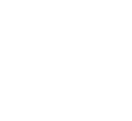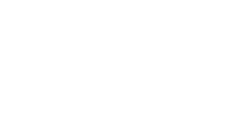Take small steps,…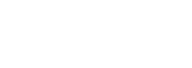so that you can tell which change broke the code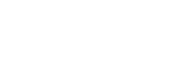When your program becomes too complicated, take a break to make it do the same thing more simply instead of making it do more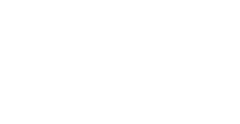## Day 3.1 – Functions and iteration

Refactoring into Functions
Iteration Notes

## Day 3.2 – Arranging items, sin and cos

Arranging Items in a Grid
Random Elements in a Grid
Arranging Items in a Circle or Spiral

In-class instructor sketches:

## Day 4.1 – Functions, Scope, Shadowing

Fruitful Functions (return)
Techniques for Exploring Parameter Spaces

## Day 4.2 – `random()`, `sin()`, and `noise()`

Code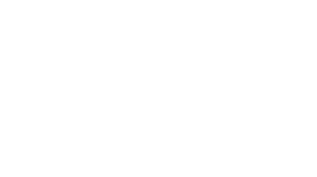## Day 5.1 – Objects

Code
``````let ball1 = {
x: 50,
y: 100,
color: "red",
};

let ball2 = {
x: 350,
y: 200,
color: "blue",
}

function setup() {
createCanvas(windowWidth, windowHeight);
}

function draw() {
background(200);

move(ball1, mouseX, mouseY);
drawBall(ball1);

move(ball2, width - mouseX, mouseY);
drawBall(ball2);
}

function drawBall(ball) {
fill(ball.color);
circle(ball.x, ball.y, 40);
}

function move(ball, targetX, targetY) {
ball.x = lerp(ball.x, targetX, 0.1);
ball.y = lerp(ball.y, targetY, 0.1);
}``````

## Day 5.2 – Classes && OOP

Code
``````let ball1, ball2;

function setup() {
createCanvas(windowWidth, windowHeight);
ball1 = new Ball(50, 50, "red");
ball2 = new Ball(350, 200, "blue");
}

function draw() {
background(200);
ball1.move(mouseX, mouseY);
ball1.draw();
ball2.move(width - mouseX, mouseY);
ball2.draw();
}

class Ball {
constructor(x, y, c) {
this.x = x;
this.y = y;
this.color = c;
}

draw() {
fill(this.color);
circle(this.x, this.y, 40);
}

// method = function that is a property of an instance of a class
move(targetX, targetY) {
this.x = lerp(this.x, targetX, 0.1);
this.y = lerp(this.y, targetY, 0.1);
}
}``````
``````let ball1, ball2;

function setup() {
createCanvas(windowWidth, windowHeight);
angleMode(DEGREES);

ball1 = new Ball(50, 50, "red");
ball2 = new Spiral(350, 20000, "green");
}

function draw() {
background(200);

ball1.move(mouseX, mouseY);
ball1.draw();

ball2.move(width - mouseX, mouseY);
ball2.draw();
}

class Ball {
constructor(x, y, c) {
this.x = x;
this.y = y;
this.color = c;
}

draw() {
fill(this.color);
}

// method = function that is a property of an instance of a class
move(targetX, targetY) {
this.x = lerp(this.x, targetX, 0.1);
this.y = lerp(this.y, targetY, 0.1);
}
}

class Spiral {
constructor(x, y, c) {
this.x = x;
this.y = y;
this.color = c;
}

draw() {
push();
translate(this.x, this.y);
fill(this.color);
for (let angle = 0; angle < 360; angle += 10) {
let r = map(angle, 0, 360, 0, this.radius);
let x = r * cos(angle);
let y = r * sin(angle);
circle(x, y, 5);
}
pop();
}

// method = function that is a property of an instance of a class
move(targetX, targetY) {
this.x = lerp(this.x, targetX, 0.1);
this.y = lerp(this.y, targetY, 0.1);
}
}``````

### Naming Conventions

Class naming conventions:

• Uppercase: `Dog` not `dog`
• Singular: `Dog` not `Dogs`

Method naming conventions:

• Don't include the class name: `Dog.speak()` not `Dog.dogSpeak()` or `Dog.speakDog()`
• CamelCase: Dog.rollOver() not Dog.roll_over(). (Unless you are modifying a sketch that already uses the `snake_case` convention. Consistency within a file is more important than consistency with a style guide.)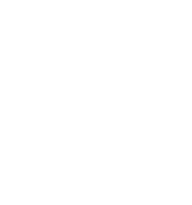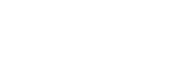## Day 5.R – OOP continued

### Old-Style Class Definitions

These are three ways of defining classes. Use the first (modern class definition). You may see the other two in older examples and tutorials, or code written by people who learned JavaScript from older tutorials.

These ways of defining a class all do the same thing*. They each define a class that is instantiated using `let ball1 = new Ball(100, 150, "red")` . Each instance has properties `x`, `y`, `radius`, and `color`,. The class has methods `draw()` and `move()`.

Modern class definition – use this

``````class Ball {
constructor(x, y, c) {
this.x = x;
this.y = y;
this.color = c;
}

draw() {
fill(this.color);
}

move(targetX, targetY) {
this.x = lerp(this.x, targetX, 0.1);
this.y = lerp(this.y, targetY, 0.1);
}
}

let b1 = new Ball(10, 20, "red");
\b1.draw();``````

Old-style: Functions

``````function Ball(x, y, c) {
this.x = x;
this.y = y;
this.color = c;

this.draw = function() {
fill(this.color);
}

this.move = function(targetX, targetY) {
this.x = lerp(this.x, targetX, 0.1);
this.y = lerp(this.y, targetY, 0.1);
}
}

let b1 = new Ball(10, 20, "red");
b1.draw();``````

Old-style: Prototype chain

``````function Ball(x, y, c) {
this.x = x;
this.y = y;
this.color = c;
}

Ball.prototype.draw = function() {
fill(this.color);
}

Ball.prototype.move = function(targetX, targetY) {
this.x = lerp(this.x, targetX, 0.1);
this.y = lerp(this.y, targetY, 0.1);
}
}

let b1 = new Ball(10, 20, "red");
b1.draw();``````

* There are subtle differences between the modern class definition, the function-based class definition, and the prototype class definition. These differences are unlikely to matter to you in practice.

## Day 6.1

### Arrays

You should be able to do the following things to an Array:

• Create a new Array. For example, create an Array that contains the three items `"Beijing"`, `"Shanghai"`, and `"Amherst"`.
• Get the nth element from an Array. For example, if `cities` has the value `["Beijing", "Shanghai", "Amherst"]`, retrieve the second element `cities` (which is `"Shanghai"`).
• Use `for(;;)` and `for (let…of…)` to iterate over the elements of an Array.
• Replace an element within an Array. For example, if `cities` has the value `["Beijing", "Shanghai", "Amherst"]`, modify it so that its value is `["Beijing", "Shanghai", "Xi'an"]`
• Add an element to the end of an Array. For example, if `cities` has the value above, modify it so that its value is `["Beijing", "Shanghai", "Amherst", "Xi'an"]`.

By the time of the midterm, you should also be able to:

• Find the position of an element within an Array. For example, if `cities` has the value `["Beijing", "Shanghai", "Amherst", "Xi'an"]`, find the value 2 such that `cities === "Amherst"`.
• Find the first element in an Array that satisfies a certain property. For example, given the property "starts with a vowel", find `"Amherst"`.
• Find all the elements within an Array that satisfy a certain property. For example, given the property "begins with the letter 'X'" and the Array ue `["Beijing", "Shanghai", "Xiamen", "Xi'an"]`, find the elements `"["Xiamen", "Xi'an"]`.

(There will not be a midterm exam. This timeframe is guidance for a pace of learning. There may be additional quizzes that cover this material, even after we are done with "Array week".)

## Day 6.2

Computation

Variables

Values, Types, and Expressions

Functions

Control Flow (`if`, `while`)

Iteration (repetition)

Array

Object

Object-Oriented Programming (classes and instances)

Workflow, Practices, & Techniques

Debugging

Iterative Development

Exploratory Programming

Bottom Up vs. Top Down

Refactoring to Functions, Classes

Project Planning & Management

Learning from Mistakes

Edit-Run-Debug-Modify Cycle

Layers

Sequencing

Tools

OpenProcessing

JavaScript Console

Glitch

Visual Studio Code

Domains

Creative Coding

Shapes

Colors

Lines and Curves

Transformations

Sound (Audio)

Images

Text and Fonts

Data (JSON)

HTML & CSS

WebGL (3D)

WebCam and PoseNet

Computational Biology (not covered)

Codons

Nucleotides

Sequences

Genes

### What's Left (The Big Picture)

• Computation – patterns of use
• Workflow – how to debug
• Tools – formatting, refactoring, Glitch, Visual Studio Code
• Domain concepts (in orange above)
• More projects
• Practice practice practice!

### Workflow Tip

Setting up a coding environment – have everything at your fingertips. I currently use a combination of Notion, and browser bookmarks, for this. Previously I've used Google docs, and HTML documents.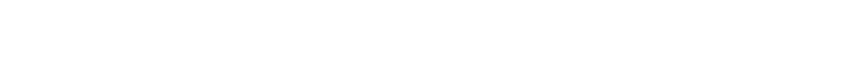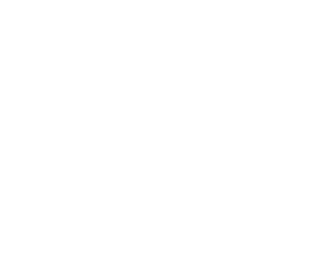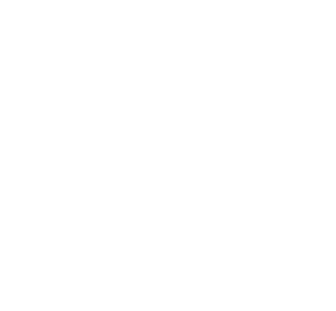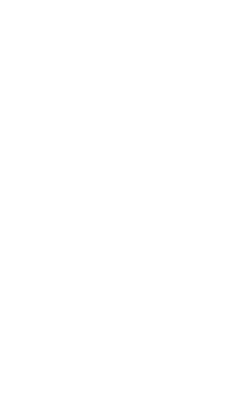### Code – Freezing Animations

Three ways of freezing an animation (of keeping `draw()` from being called again and again):

``````function setup() {
createCanvas(windowWidth, windowHeight);
background(100);
// drawing code goes here
}``````

``````function setup() {
createCanvas(windowWidth, windowHeight);
}

function draw() {
background(100);
// drawing code goes here
noLoop();
}

function mousePressed() {
loop();
}``````
``````function setup() {
createCanvas(windowWidth, windowHeight);
noLoop();
}

function draw() {
background(100);
// drawing code goes here
}

function mousePressed() {
loop();
}``````

### Code – Two Array Iteration Methods

Two ways of iterating over all the items in an Array:

``````let teams = [
"Interactive Music Visualization",
"Animal Farm",
"Smash",
"May The Force Be With You",
"Interactive Game",
"Oasis in the Distance",
];

function setup() {
createCanvas(windowWidth, windowHeight);
for (let i = 0; i < teams.length; i++) {
let team = teams[i];
console.info(team);
}
}``````
``````let teams = [
"Interactive Music Visualization",
"Animal Farm",
"Smash",
"May The Force Be With You",
"Interactive Game",
"Oasis in the Distance",
];

function setup() {
createCanvas(windowWidth, windowHeight);
for (let team of teams) {
console.info(team);
}
}``````

### Code – Shuffling Team Names

Code: Shuffle Team Names
``````let teams = [
"Interactive Music Visualization",
"Animal Farm",
"Smash",
"May The Force Be With You",
"Interactive Game",
"Oasis in the Distance",
];

function setup() {
createCanvas(windowWidth, windowHeight);
}

function draw() {
background(100);

textSize(40);
fill('white');
let y = 40;

teams = shuffle(teams);

for (let team of teams) {
text(team, 10, y);
y += 45;
}
noLoop();
}

function mousePressed() {
loop();
}``````

### Code – Arrays of Objects

Code: Arrays of Objects

Draw a ball.

``````let x = 10;
let y = 50;
let size = 80;

function setup() {
createCanvas(windowWidth, windowHeight);
}

function draw() {
background(100);

circle(x, y, size);
}``````

Instead of using a separate variable for each of the ball's qualities, turn them into properties of an Object. The ball is represented by a single variable, whose value is an Object with multiple properties.

``````let ball = {x: 10, y: 50, size: 80};

function setup() {
createCanvas(windowWidth, windowHeight);
}

function draw() {
background(100);

circle(ball.x, ball.y, ball.size);
}``````

``````let ball1 = {x: 10, y: 50, size: 80};
let ball2 = {x: 80, y: 150, size: 60};

function setup() {
createCanvas(windowWidth, windowHeight);
}

function draw() {
background(100);

circle(ball1.x, ball1.y, ball1.size);
circle(ball2.x, ball2.y, ball2.size);
}``````

Change the code so that the two calls to `circle()` look the same. This makes the code less direct, but also sets it up to be more flexible, so that we can use Arrays and iteration in the following steps.

``````let ball1 = {x: 10, y: 50, size: 80};
let ball2 = {x: 80, y: 150, size: 60};

function setup() {
createCanvas(windowWidth, windowHeight);
}

function draw() {
background(100);

{
let ball = ball1;
circle(ball.x, ball.y, ball.size);
}

{
let ball = ball2;
circle(ball.x, ball.y, ball.size);
}
}``````

Let's collect `ball1` and `ball2` into an Array. This continues to make the code less direct, but more flexible.

``````let ball1 = {x: 10, y: 50, size: 80};
let ball2 = {x: 80, y: 150, size: 60};
let balls;

function setup() {
createCanvas(windowWidth, windowHeight);
balls = [ball1, ball2];
}

function draw() {
background(100);

{
let ball = balls;
circle(ball.x, ball.y, ball.size);
}

{
let ball = balls;
circle(ball.x, ball.y, ball.size);
}
}``````

The pattern above, where we do something with the first element of an Array and then the exact same thing for the next element of the Array, can be replaced by a `for` loop.

``````let ball1 = {x: 10, y: 50, size: 80};
let ball2 = {x: 80, y: 150, size: 60};
let balls;

function setup() {
createCanvas(windowWidth, windowHeight);
balls = [ball1, ball2];
}

function draw() {
background(100);

for (let ball of balls) {
circle(ball.x, ball.y, ball.size);
}
}``````

`ball1` and `ball2` are only used inside of `setup()`, so we can also define them there. (This makes it easier to see where these variables are used, and what code we need to change if we modify or remove them.)

``````let balls;

function setup() {
createCanvas(windowWidth, windowHeight);
let ball1 = {x: random(width), y: random(height), size: 80};
let ball2 = {x: random(width), y: random(height), size: 80};
balls = [ball1, ball2];
}

function draw() {
background(100);

for (let ball of balls) {
circle(ball.x, ball.y, ball.size);
}
}``````

Define functions `createBall()` and `drawBall()`, that create the ball objects and use their properties to draw onto the canvas:

``````let balls;

function setup() {
createCanvas(windowWidth, windowHeight);
let ball1 = createBall();
let ball2 = createBall();
let ball3 = createBall();
balls = [ball1, ball2, ball3];
}

function draw() {
background(100);

for (let ball of balls) {
drawBall(ball);
}
}

function createBall() {
let ball = {x: random(width), y: random(height), size: 80};
return ball;
}

function drawBall(ball) {
circle(ball.x, ball.y, ball.size)
}``````

We don't need the variables `ball1`, `ball2`, etc. We can create the objects "in place" as we create the Array.

``````function setup() {
createCanvas(windowWidth, windowHeight);
balls = [createBall(), createBall(), createBall()];
}``````

Now it is easy to create any number of balls (say, 30):

``````function setup() {
createCanvas(windowWidth, windowHeight);
balls = [];
for (let i = 0; i < 30; i++) {
balls.push(createBall());
}
}``````

Create a `Ball` class. Compare this to the preceding sketch.

``````let balls;

function setup() {
createCanvas(windowWidth, windowHeight);
balls = [];
for (let i = 0; i < 20; i++) {
let ball = new Ball();
balls.push(ball);
}
}``````

Add a ball when the user presses the mouse

``````let balls;

function setup() {
createCanvas(windowWidth, windowHeight);
balls = [];
for (let i = 0; i < 20; i++) {
let ball = new Ball(random(width), random(height));
balls.push(ball);
}
}

function draw() {
background(200 + 50 * cos(frameCount / 25));

for (let ball of balls) {
ball.draw();
}
}

function mousePressed() {
let ball = new Ball(mouseX, mouseY);
balls.push(ball);
}

class Ball {
constructor(x, y) {
this.x = x;
this.y = y;
this.size = 80;
}

draw() {
circle(this.x, this.y, this.size);
}
}``````

Outline the balls that are near to the mouse:

``````let balls;

function setup() {
createCanvas(windowWidth, windowHeight);
balls = [];
for (let i = 0; i < 20; i++) {
let ball = new Ball(random(width), random(height));
balls.push(ball);
}
}

function draw() {
background(200); // + 50 * cos(frameCount / 25));

// draw the balls
for (let ball of balls) {
ball.draw();
}

// outline the balls
for (let ball of balls) {
if (ball.closeToMouse()) {
ball.outline();
}
}
}

function mousePressed() {
let ball = new Ball(mouseX, mouseY);
balls.push(ball);
}

class Ball {
constructor(x, y) {
this.x = x;
this.y = y;
this.size = 80;
}

draw() {
circle(this.x, this.y, this.size);
}

closeToMouse() {
let d = dist(this.x, this.y, mouseX, mouseY);
return d < this.size * 2;
}

outline() {
push();
noFill();
strokeWeight(5);
circle(this.x, this.y, this.size + 15);
pop();
}
}``````

### Code – `closeToMouse()` (review of booleans and return values)

Several ways of writing `closeToMouse()`:

``````class Ball {
constructor() {
// …
}
closeToMouse() {
let d = dist(this.x, this.y, mouseX, mouseY);
if (d < this.size) {
return true;
} else {
return false;
}
}
draw() {
// …
}
}``````
``````	closeToMouse() {
let d = dist(this.x, this.y, mouseX, mouseY);
return d < this.size * 2;
}``````
``````	closeToMouse() {
return dist(this.x, this.y, mouseX, mouseY) < this.size * 2;
}``````

If P5.js didn't define `dist()`:

``````	closeToMouse() {
let dx = this.x - mouseX;
let dy = this.y - mouseY;
let d = sqrt(sq(dx) + sq(dy));
return d < this.size * 2;
}``````

### Code – variable scope (review)

Review: scope

This doesn't work, because `draw()` can't "see" the variables that are defined inside of `setup()`. (`setup()` gets its own dictionary while it is running. Variables defined inside of `setup()` go in this dictionary. When `setup()` returns, its dictionary disappears.)

``````let ball1 = {x: 10, y: 50, size: 80};
let ball2 = {x: 80, y: 150, size: 60};

function setup() {
createCanvas(windowWidth, windowHeight);
let balls = [ball1, ball2];
}

function draw() {
background(100);

{
let ball = ball;
circle(ball.x, ball.y, ball.size);
}

{
let ball = ball;
circle(ball.x, ball.y, ball.size);
}
}``````

``````let ball1 = {x: 10, y: 50, size: 80};
let ball2 = {x: 80, y: 150, size: 60};
let balls;

function setup() {
createCanvas(windowWidth, windowHeight);
balls = [ball1, ball2];
}

function draw() {
background(100);

{
let ball = ball;
circle(ball.x, ball.y, ball.size);
}

{
let ball = ball;
circle(ball.x, ball.y, ball.size);
}
}``````

### Code – Optional Arguments with Default Values

This is optional material. You do not need to understand or use this in this course.

Optional arguments
``````let balls;

function setup() {
createCanvas(windowWidth, windowHeight);
balls = [];
for (let i = 0; i < 20; i++) {
let ball = new Ball();
balls.push(ball);
}
}

function draw() {
background(200); // + 50 * cos(frameCount / 25));

// draw the balls
for (let ball of balls) {
ball.draw();
}

// outline the balls
for (let ball of balls) {
if (ball.closeToPoint()) {
ball.outline();
}
}
}

function mousePressed() {
let ball = new Ball(mouseX, mouseY);
balls.push(ball);
}

class Ball {
constructor(x = random(width), y = random(height)) {
this.x = x;
this.y = y;
this.size = 80;
}

draw() {
circle(this.x, this.y, this.size);
}

closeToPoint(x = mouseX, y = mouseY) {
let d = dist(this.x, this.y, x, y);
return d < this.size * 2;
}

outline() {
push();
noFill();
strokeWeight(5);
circle(this.x, this.y, this.size + 15);
pop();
}
}``````

## Day 6.R – Array.select and Particles

``````let balls;

function setup() {
createCanvas(windowWidth, windowHeight);
balls = [];
for (let i = 0; i < 20; i++) {
let ball = new Ball(random(width), random(height));
balls.push(ball);
}
}

function draw() {
background(200); // + 50 * cos(frameCount / 25));

// draw the balls
for (let ball of balls) {
ball.draw();
}
}

function mousePressed() {
let ball = new Ball(mouseX, mouseY);
balls.push(ball);
}

class Ball {
constructor(x, y) {
this.x = x;
this.y = y;
this.size = 80;
}

draw() {
circle(this.x, this.y, this.size);
}

closeToMouse() {
let d = dist(this.x, this.y, mouseX, mouseY);
return d < this.size * 2;
}
}``````